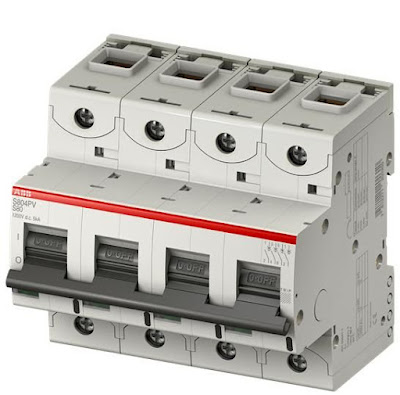DC Circuit Breaker | Source: ABB

For the correct sizing of a circuit breaker in a direct current network, some electrical parameters which characterize the device itself must be evaluated.

Rated operational voltage Ve

This represents the value of the application voltage of the equipment and to which all the other equipment parameters are referred.

Rated uninterrupted current Iu

This represents the value of current that the equipment can carry for an indefinite time (uninterrupted duty). This parameter is used to define the size of the circuit breaker.

Related Article:

Rated current In

This represents the value of the current of the trip unit mounted on the circuit breaker and determines the protected characteristic of the circuit breaker itself according to the available settings of the trip unit. This current is often referred to as the rated current of the load protected by the circuit breaker itself.

Rated ultimate short-circuit breaking capacity Icu

The rated ultimate short-circuit breaking capacity of a circuit breaker is the maximum short-circuit current value which the circuit breaker can break twice (in accordance with the sequence O – t – CO) at the corresponding rated operational voltage. After the opening and closing sequence the circuit breaker is not required to carry its rated current.

Rated service short-circuit breaking capacity Ics

The rated service short-circuit breaking capacity of a circuit breaker is the maximum short-circuit current value which the circuit breaker can break three times, in accordance with a sequence of opening and closing operations (O - t - CO - t – CO), at a defined rated operational voltage (Ve) and at a defined time constant (for direct current). After this sequence, the circuit breaker is required to carry its rated current.

Rated short-time withstands current Icw

The rated short-time withstand current is the current that the circuit breaker in the closed position can carry during a specified short time under prescribed conditions of use and behavior. The circuit breaker shall be able to carry this current during the associated short-time delay in order to ensure discrimination between the circuit breakers in series.

### Sizing Circuit Breakers in DC Electrical System

In the previous pages, the main electrical characteristics needed to choose the correct circuit breaker have been defined so that protection of the plant is guaranteed. To size the circuit breaker, it is necessary to know the following characteristics of the network:

1. The type of network (see:  Type of DC Network: Network with the middle point of the supply source connected to ground and other DC Network System), to define the connection of the circuit breaker poles according to the possible fault conditions;
2. The rated voltage of a plant (Vn), to define the operational voltage (Ve) depending on the pole connection by verifying the relation: Vn≤ Ve;
3. The short-circuit current at the installation point of the circuit breaker (Ik), to define the circuit breaker version (depending on the connection of the poles) by verifying the relation Ik ≤ Icu (at the reference rated operational voltages Ve).
4. The rated current absorbed by the load (Ib), to define the rated current (In) of the thermal-magnetic trip unit or of the DC electronic trip unit by verifying the relation Ib≤ In.

The following diagram summarizes the choices for correct sizing of the circuit breaker in relation to the characteristics of the plant.Sizing Circuit Breaker

Source:

• ABB Circuit Breakers for Direct Current Applications | pp. 20-23
• Publisher: ABB